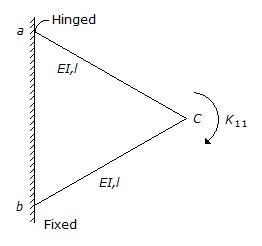# Civil Engineering - GATE Exam Questions

### Exercise :: GATE Exam Questions - Section 4

16.

Rotational stiffness-coefficient, K11 for the frame having two members of equal EI/l is given by :A. 5 EI/l B. 6 EI/l C. 7 EI/l D. 8 EI/l

Explanation:

No answer description available for this question. Let us discuss.

17.

The plan of a survey plotted to a scale of 10 m to 1 cm is reduced in such a way that a line originally 10 cm long now measures 9 cm. The area of the reduced plan is measured as 81 cm2. The actual (m2) of the survey is

 A. 10000 B. 6561 C. 1000 D. 656

Explanation:

No answer description available for this question. Let us discuss.

18.

Width of carriage way for a single lane is recommended to be

 A. 7.5 m B. 7.0 m C. 3.75 m D. 5.5 m

Explanation:

No answer description available for this question. Let us discuss.

19.

The shape of the cross-section, which has the largest shape factor, is

 A. rectangular B. I-section C. diamond D. solid circular

Explanation:

No answer description available for this question. Let us discuss.

20.

The unit of dynamic viscosity of a fluid is

 A. m2/s B. Ns/m2 C. Pa s/m2 D. kg s2/m2